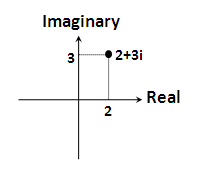Roger's

This is the way.

``````代数式,例如:2+3i

``````

i=√-1 在 2+3i 中,2 通常被称为复数的实部，3i 被称为复数的虚部``````极坐标形式为(r,θ)

``````

``````(r,θ) = r*cos(θ) + r*sin(θ)*i

``````

`F = |F| e^iθ = |F|(cosθ+isinθ) = a+ib`

#### 乘除运算

e^jθ 旋转因子 e^jθ = cosθ+jsinθ = (1,θ)

``````θ = π/2时，e^j(π/2) = cos(π/2)+jsin(π/2) = +j
θ = -π/2时,e^j(-π/2) = cos(-π/2)+jsin(-π/2) = -j
θ = 0时,e^j0 = 1
θ = +-π时,e^(+-jπ) = cos(+-π)+jsin(+-π) = -1
``````

* 邮箱仅用于回复提醒，不会被公开